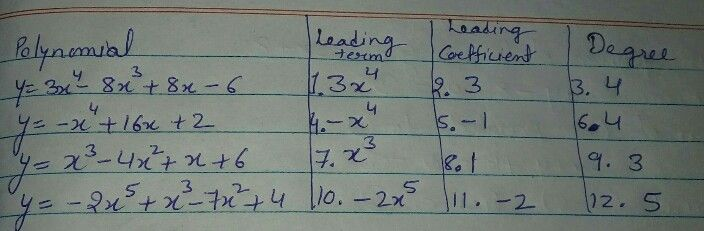Symbol
ProblemComplete Me! $Diractions:$ Complete the table below. If the given is a polynomial function, give the degree, leading coefficient and its constant term. Polynomial Function Leading Leading Term Coefficient Degree $y=3x^{4}-8x^{3}+8x-6$ $1$ $2.$ $3.$ $y=-x^{4}+16x+2$ $4$ $5$ $6$ $y=x^{3}-4x^{2}+x+6$ $7$ $8$ $9.$ $y=-2x^{5}+x^{3}-x^{2}+4$ $10$ $11$ $12$
10th-13th grade
Other
Search count: 112
Question content
please help me solve this problem teacher pleaseee..For the exam.
SolutionQanda teacher - ANILKUMAR